Home » A Beginner-Friendly Guide to PyTorch and How it Works from Scratch

# A Beginner-Friendly Guide to PyTorch and How it Works from Scratch

## Overview

• What is PyTorch? How can you get started with it from scratch? We’ll cover all of that in this article
• PyTorch is one of the most popular deep learning libraries right now
• We’ll also take up a case study and use PyTorch to understand its practice applications

## Introduction

Every once in a while, there comes a library or framework that reshapes and reimagines how we look at the field of deep learning. The remarkable progress a single framework can bring about never ceases to amaze me.

I can safely say PyTorch is on that list of deep learning frameworks. It has helped accelerate the research that goes into deep learning models by making them computationally faster and less expensive (a data scientist’s dream!).

I’ve personally found PyTorch really useful for my work. I delve heavily into the arts of computer vision and find myself leaning on PyTorch’s flexibility and efficiency quite often.So in this article, I will guide you on how PyTorch works, and how you can get started with it today itself. We’ll cover everything there is to cover about this game-changing deep learning framework and also take up a really cool case study to see PyTorch in action.

Part II and III of this series are now live, and you can access them here:

If you prefer to approach learning PyTorch and the below concepts in a structured format, you can enrol for this free course and follow the concepts chapter-wise: PyTorch Course

1. Getting Started with PyTorch
2. Basics of PyTorch
1. Introduction to Tensors
2. Mathematical Operations
3. Matrix Initialization and Matrix Operations
3. Common PyTorch Modules
2. Optim
3. nn
4. Building a Neural Network from Scratch in PyTorch
5. Solving an Image Classification Problem using PyTorch
6. What’s Next?

## Getting Started with PyTorchPyTorch is a Python-based library that provides maximum flexibility and speed.

I’ve found PyTorch to be as simple as working with NumPy – and trust me, that is not an exaggeration.

You will figure this out really soon as we move forward in this article. But before we dive into the nuances of PyTorch, let’s look at some of the key features of this framework which make it unique and easy to use.

### TorchScript

PyTorch TorchScript helps to create serializable and optimizable models. Once we train these models in Python, they can be run independently from Python as well. This helps when we’re in the model deployment stage of a data science project.

So, you can train a model in PyTorch using Python and then export the model via TorchScript to a production environment where Python is not available. We will discuss model deployment in more detail in the later articles of this series.

### Distributed Training

PyTorch also supports distributed training which enables researchers as well as practitioners to parallelize their computations. Distributed training makes it possible to use multiple GPUs to process larger batches of input data. This, in turn, reduces the computation time.

### Python Support

PyTorch has a very good interaction with Python. In fact, coding in PyTorch is quite similar to Python. So if you are comfortable with Python, you are going to love working with PyTorch.

### Dynamic Computation Graphs

PyTorch has a unique way of building neural networks. It creates dynamic computation graphs meaning that the graph will be created on the fly: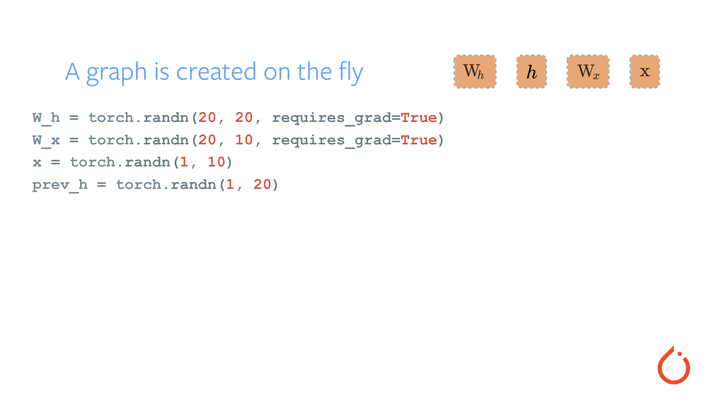And this is just skimming the surface of why PyTorch has become such a beloved framework in the data science community.

Right – now it’s time to get started with understanding the basics of PyTorch. So make sure you install PyTorch on your machine before proceeding. The latest version of PyTorch (PyTorch 1.2) was released on August 08, 2019 and you can see the installation steps for it using this link.

## Basics of PyTorch

Remember how I said PyTorch is quite similar to Numpy earlier? Let’s build on that statement now. I will demonstrate basic PyTorch operations and show you how similar they are to NumPy.

In the NumPy library, we have multi-dimensional arrays whereas in PyTorch, we have tensors. So, let’s first understand what tensors are.

### Introduction to Tensors

Tensors are multidimensional arrays. And PyTorch tensors are similar to NumPy’s n-dimensional arrays. We can use these tensors on a GPU as well (this is not the case with NumPy arrays). This is a major advantage of using tensors.

PyTorch supports multiple types of tensors, including:

1. FloatTensor: 32-bit float
2. DoubleTensor: 64-bit float
3. HalfTensor: 16-bit float
4. IntTensor: 32-bit int
5. LongTensor: 64-bit int

Now, let’s look at the basics of PyTorch along with how it compares against NumPy. If you are familiar with other deep learning frameworks, you must have come across tensors in TensorFlow as well. In fact, you are welcome to implement the following tasks in Tensorflow too and make your own comparison of PyTorch vs. TensorFlow!

We’ll start by importing both the NumPy and the Torch libraries:

Now, let’s see how we can assign a variable in NumPy as well as PyTorch: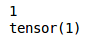Let’s quickly look at the type of both these variables: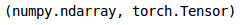Type here confirms that the first variable (a) here is a NumPy array whereas the second variable (b) is a torch tensor.

Next, we will see how to perform mathematical operations on these tensors and how it is similar to NumPy’s mathematical operations.

### Mathematical operations

Do you remember how to perform mathematical operations on NumPy arrays? If not, let me quickly recap that for you.

We will initialize two arrays and then perform mathematical operations like addition, subtraction, multiplication, and division, on them: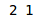These are the two NumPy arrays we have initialized. Now let’s see how we can perform mathematical operations on these arrays: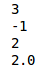Let’s now see how we can do the same using PyTorch on tensors. So, first, let’s initialize two tensors: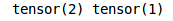Next, perform the operations which we saw in NumPy: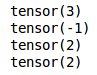Did you see the similarities? The codes are exactly the same to perform the above mentioned mathematical operations in both NumPy and PyTorch.

Next, let’s see how to initialize a matrix as well as perform matrix operations in PyTorch (along with, you guessed it, it’s NumPy counterpart!).

### Matrix Initialization

Let’s say we want a matrix of shape 3*3 having all zeros. Take a moment to think – how can we do that using NumPy?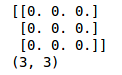Fairly straightforward. We just have to use the zeros() function of NumPy and pass the desired shape ((3,3) in our case), and we get a matrix consisting of all zeros. Let’s now see how we can do this in PyTorch: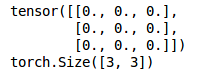Similar to NumPy, PyTorch also has the zeros() function which takes the shape as input and returns a matrix of zeros of a specified shape. Now, while building a neural network, we randomly initialize the weights for the model. So, let’s see how we can initialize a matrix with random numbers: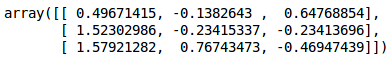We have specified the random seed at the beginning here so that every time we run the above code, the same random number will generate. The random.randn() function returns random numbers that follow a standard normal distribution.

But let’s not get waylaid by the statistics part of things. We’ll focus on how we can initialize a similar matrix of random numbers using PyTorch: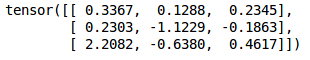This is where even more similarities with NumPy crop up. PyTorch also has a function called randn() that returns a tensor filled with random numbers from a normal distribution with mean 0 and variance 1 (also called the standard normal distribution).

Note that we have set the random seed here as well just to reproduce the results every time you run this code. So far, we have seen how to initialize a matrix using PyTorch. Next, let’s see how to perform matrix operations in PyTorch.

### Matrix Operations

We will first initialize two matrices in NumPy:

Next, let’s perform basic operations on them using NumPy: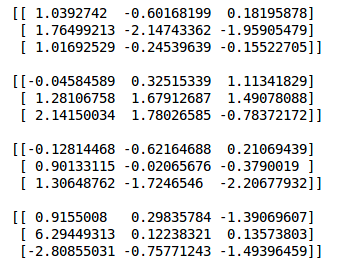Matrix transpose is one technique which is also very useful while creating a neural network from scratch. So let’s see how we take the transpose of a matrix in NumPy: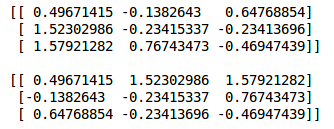The transpose() function of NumPy automatically returns the transpose of a matrix. How does this happen in PyTorch? Let’s find out: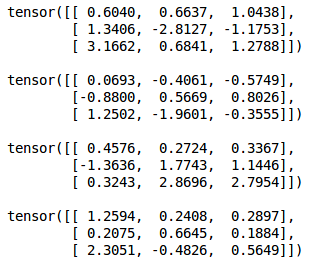Note that the .mm() function of PyTorch is similar to the dot product in NumPy. This function will be helpful when we create our model from scratch in PyTorch. Calculating transpose is also similar to NumPy: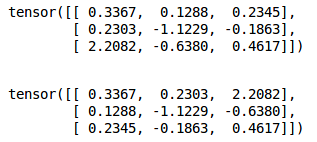Next, we will look at some other common operations like concatenating and reshaping tensors. From this point forward, I will not be comparing PyTorch against NumPy as you must have got an idea of how the codes are similar.

### Concatenating Tensors

Let’s say we have two tensors as shown below: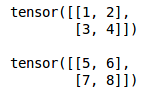What if we want to concatenate these tensors vertically? We can use the below code: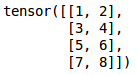As you can see, the second tensor has been stacked below the first tensor. We can concatenate the tensors horizontally as well by setting the dim parameter to 1: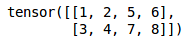### Reshaping Tensors

Let’s say we have the following tensor: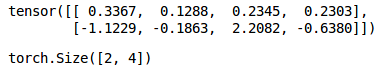We can use the .reshape() function and pass the required shape as a parameter. Let’s try to convert the above tensor of shape (2,4) to a tensor of shape (1,8):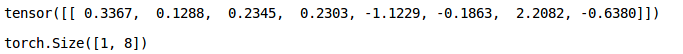Awesome! PyTorch also provides the functionality to convert NumPy arrays to tensors. You can use the below code to do it: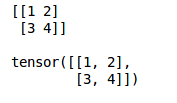With me so far? Good – let’s move on and dive deeper into the various aspects of PyTorch.

## Common PyTorch Modules

PyTorch uses a technique called automatic differentiation. It records all the operations that we are performing and replays it backward to compute gradients. This technique helps us to save time on each epoch as we are calculating the gradients on the forward pass itself.

Let’s look at an example to understand how the gradients are computed: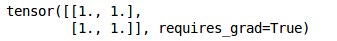Here, we have initialized a tensor. Specifying requires_grad as True will make sure that the gradients are stored for this particular tensor whenever we perform some operation on it. Let’s now perform some operations on the defined tensor: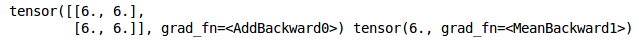First of all, we added 5 to all the elements of this tensor and then taken the mean of that tensor. We will first manually calculate the gradients and then verify that using PyTorch. We performed the following operations on a:

b = a + 5

c = mean(b) = Σ(a+5) / 4

Now, the derivative of c w.r.t. a will be ¼ and hence the gradient matrix will be 0.25. Let’s verify this using PyTorch: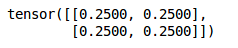As expected, we have the gradients.

The autograd module helps us to compute the gradients in the forward pass itself which saves a lot of computation time of an epoch.

### Optim Module

The Optim module in PyTorch has pre-written codes for most of the optimizers that are used while building a neural network. We just have to import them and then they can be used to build models.

Let’s see how we can use an optimizer in PyTorch:

Above are the examples to get the ADAM and SGD optimizers. Most of the commonly used optimizers are supported in PyTorch and hence we do not have to write them from scratch. Some of them are:

• SGD
• ASGD (Averaged Stochastic Gradient Descent)
• RMSprop
• Rprop (resilient backpropagation)

### nn Module

The autograd module in PyTorch helps us define computation graphs as we proceed in the model. But, just using the autograd module can be low-level when we are dealing with a complex neural network.

In those cases, we can make use of the nn module. This defines a set of functions, similar to the layers of a neural network, which takes the input from the previous state and produces an output.

We will use all these modules and define our neural network to solve a case study in the later sections. For now, let’s build a neural network from scratch that will help us understand how PyTorch works in a practical way.

## Building a Neural Network from Scratch in PyTorch

I hope you are comfortable with building a neural network from scratch using NumPy. If not, I highly recommend you go through this article.

Alright – time to get started with neural networks! This is going to be a lot of fun so let’s get right down to it. We will first initialize the input and output: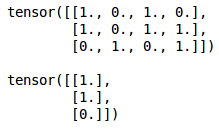Next, we will define the sigmoid function which will act as the activation function and the derivative of the sigmoid function which will help us in the backpropagation step:

Next, initialize the parameters for our model including the number of epochs, learning rate, weights, biases, etc.:

Here we have randomly initialized the weights and biases using the .randn() function which we saw earlier. Finally, we will create a neural network. I am taking a simple model here just to make things clear. There is a single hidden layer and an input and an output layer in the model:

In the forward propagation step, we are calculating the output and finally, in the backward propagation step, we are calculating the error. We will then update the weights and biases using this error.

Let’s now look at the output from the model: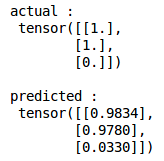So, the target is 1, 1, 0 and the predicted values from the model are 0.98, 0.97 and 0.03. Not bad at all!

This is how we can build and train a neural network from scratch in PyTorch. Let’s now take things up a notch and dive into a case study. We will try to solve that case study using the techniques we have learned in this article.

## Solving an Image Classification Problem using PyTorch

You’re going to love this section. This is where all our learning will culminate in a final neural network model on a real-world case study, and we will see how the PyTorch framework builds a deep learning model.

Our task is to identify the type of apparel by looking at a variety of apparel images. It’s a classic image classification problem using computer vision. This dataset, taken from the DataHack Platform, can be downloaded here.

There are a total of 10 classes in which we can classify the images of apparels:

 Label Description 0 T-shirt/top 1 Trouser 2 Pullover 3 Dress 4 Coat 5 Sandal 6 Shirt 7 Sneaker 8 Bag 9 Ankle boot

There are 70,000 images, out of which 60,000 are in the training set and the remaining 10,000 in the test set. All the images are grayscale images of size (28*28).

The dataset contains two folders – one each for the training set and the test set. In each folder, there is a .csv file that has the id of the image and its corresponding label and a folder containing the images for that particular set.

Let’s now get started with the code! We will first import the required libraries: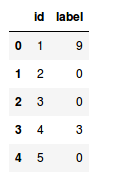id here represents the name of the image (we just have to add .png as the images are in png format) and the label is the corresponding class of that particular image.

Let’s now plot an image to get a better understanding of how our data looks. We will randomly select an image and plot it. So, let’s first create a random number generator:

Plot an image: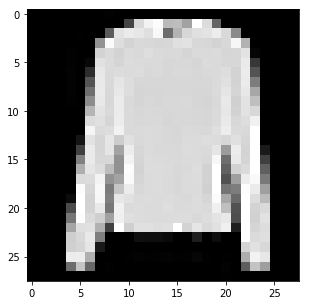This is a random image from our dataset and gives us an idea of what all the other images look like. Next, we will load all the training images using the train.csv file. We will use a for loop to read all the images from the training set and finally store them as a NumPy array: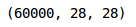So, there are 60,000 images in the training set each of shape 28 x 28. We will be making a simple neural network which takes a one-dimensional input and hence we have to flatten these two-dimensional images into a single dimension: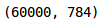We have reshaped the images to a single dimension. So far, we have created the input set but we also need the target to train the model, right? So, let’s go ahead and create that:

### Training the model

Let’s create a validation set to evaluate how well our model will perform on unseen data: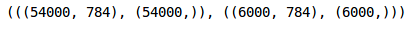We have taken 10 percent of the training data in the validation set.

Now, it’s time to define our model. We will first import the Torch package and the required modules:

Next, define the parameters like the number of neurons in the hidden layer, the number of epochs, and the learning rate:

Finally, let’s build the model! For now, we will have a single hidden layer and choose the loss function as cross-entropy. We will be using the Adam optimizer here. Remember that there are other parameters of our model and you can change them as well.

Let’s now train the model for a specified number of epochs and save the training and validation loss for each epoch: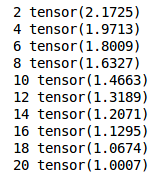Here, I have printed the training losses after every second epoch and we can see that the loss is decreasing. Let’s now plot the training and validation loss to check whether they are in sync or not: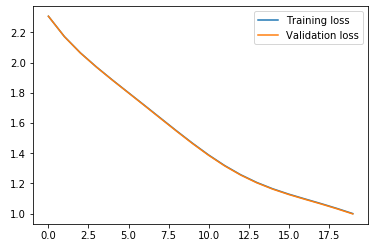Perfect! We can see that the training and validation losses are in sync and the model is not overfitting. Let’s now check how accurate our model is on predicting the classes for both training and validation sets. We’ll start by looking at the training accuracy: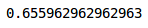We got an accuracy of above 65% on the training set. Let’s check for the validation set as well: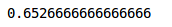We have an almost similar performance on the validation set. Note that even though we have used a very simple architecture with just one hidden layer, the performance is pretty good.

You can try to increase the number of hidden layers or play with other model parameters like the optimizer function, the number of hidden units, etc. and try to improve the performance further.

### Getting predictions

Finally, let’s load the test images, make predictions on them and submit the predictions on the competition page. We will have to preprocess the test images in a similar way that we did for the training images: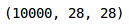Let’s convert these images to a 1-d array now: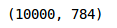Finally, we will make predictions for these images:

Great, we now have the predictions. We will now save these predictions in the sample submission file: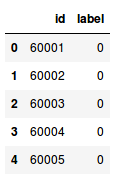Replace these labels with the predictions that we got from the model for test images: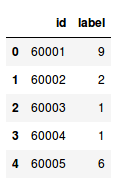Save this sample submission file and make a submission on the competition page:

After submitting these predictions, we get an accuracy of 64.625% on the leaderboard. You can use this accuracy as a benchmark and try to improve on this by playing around with the parameters of the above model.

## End Notes

In this article, we understood the basic concepts of PyTorch including how it’s quite intuitively similar to NumPy. We also saw how to build a neural network from scratch using.

We then took a case study where we solved an image classification problem and got a benchmark score of around 65% on the leaderboard. I encourage you to try and improve this score by changing different parameters of the model, including the optimizer function, increasing the number of hidden layers, tuning the number of hidden units, etc.

This article is the start of our journey with PyTorch. In the upcoming article, we will learn how to build convolutional neural networks in PyTorch, model checkpointing techniques, and how to deploy trained deep learning models using PyTorch. So stay tuned!

As always, if you have any doubts related to this article, feel free to post them in the comments section below. And make sure you check out our course if you’re interested in computer vision and deep learning:

You can also read this article on Analytics Vidhya's Android APP•Anna says:

Awesome post, thanks for sharing.

•Pulkit Sharma says:

•Rajat says:

I don’t how to thank you Pulkit. So much informative and well-explained article. Keep up the good work.

•Pulkit Sharma says:

•Hayato Kawajiri says:

Amazing article, broadens as once seemingly narrow concept and gives food for thought. Thank you.

•Pulkit Sharma says:

Thank you for your feedback Hayato!

•purna says:

I think “output = sigmoid(output_layer_input1)” , this should be ” output = sigmoid(output_layer_input). please correct me if I am wrong. Thank you

•Pulkit Sharma says:

Hi Purna,
Yes you are correct. Thank you for pointing it out. I have updated the same in the article.

•Vol says:

This is a very well written post. Excellent attempt to communicate concepts for beginners.

Please take a look at the derivative of sigmoid function again.

https://math.stackexchange.com/questions/78575/derivative-of-sigmoid-function-sigma-x-frac11e-x

•Pulkit Sharma says:

Hi,
That’s a good catch. Thank you for pointing it out. The derivative of a sigmoid function is sigmoid(x) * [1 – sigmoid(x)]. I have updated the same in the article.